Previous: 3.2.2 Critique and Modifications Up: 3.2 Maximum Entropy Closure Next: 3.3 Higher Order Statistics

## 3.2.3 Diffusion Closure

The diffusion closure is a linearized version of the maximum entropy closure. We rewrite a distribution function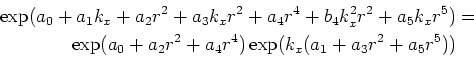(3.11)

and assume that the coefficients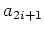are small, that is, we stay in the diffusion limit. Then we linearize the second factor and get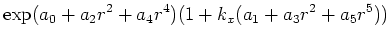(3.12)

which is of the linear-isotropic type. Given the even moments, the parameters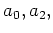andcan be determined. However, not for every combination of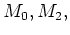andfrom a distribution function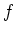we can find such parameters. In particular, it can be shown, that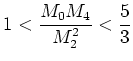(3.13)

which limits the range of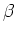, whereis defined as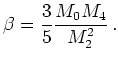(3.14)

In Equation 3.13 the value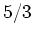comes from a Gaussian distribution. The value of 1 is reached from a distribution function of the form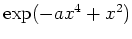in the limit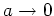.

Likewise one can show that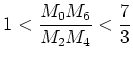(3.15)

must hold. Our implementation of the diffusion closure uses one lookup table forand one for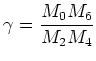(3.16)

both parameterized by the single parameter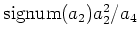. From the study of Monte Carlo data we know that the range of parametersand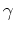in the results largely exceeds the range ofandgiven by Equations 3.13 and 3.15.